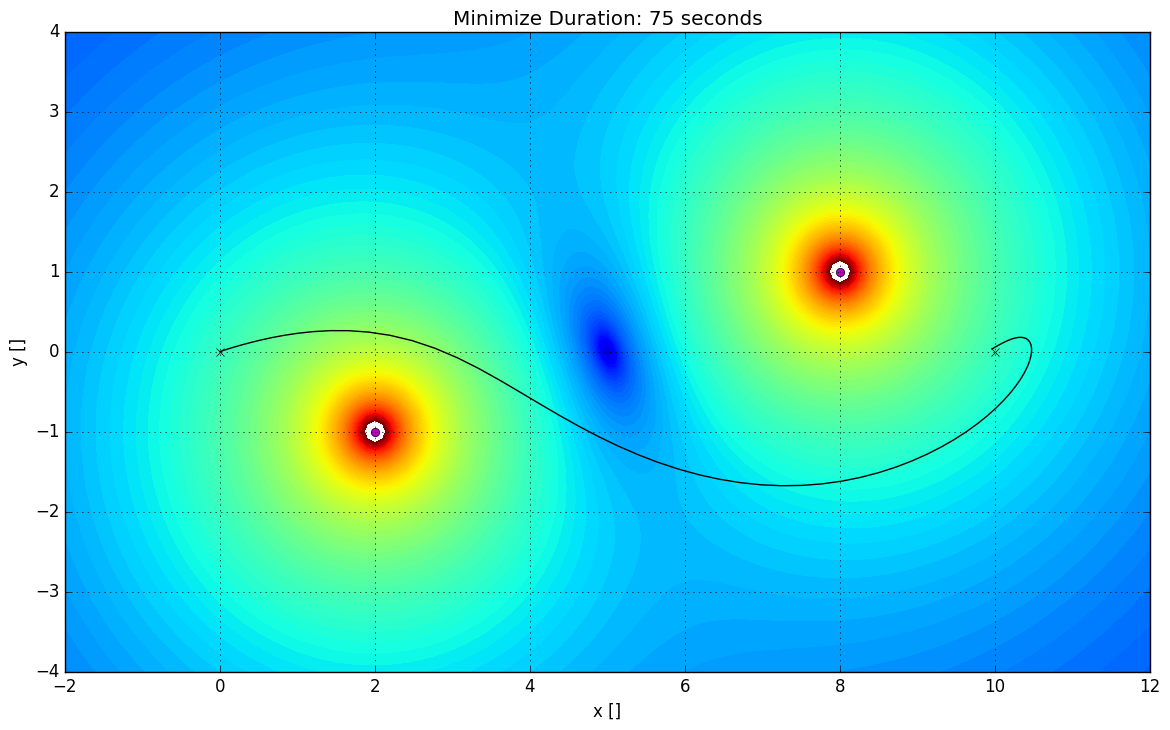# Optimization of Trajectories through Dynamical Gravitational Systems¶

## 1. Introduction¶

Our project focused on finding the optimal path a space probe could take while traveling in the inner solar system. The model we created accounts for the gravity that large celestial objects produce, as well as their orbits. The inspiration for our problem comes from the Nasa Missions Voyager and Mariner. The Voyager missions launched in 1977 (“Voyager - Planetary,” 2015) and the Mariner Missions occurred throughout the 1960s and early 1970s ("Mariner Missions," n.d.). These missions involved space probes traveling throughout the inner and outer Solar System, visiting multiple planets and studying each with various onboard instruments. Here's a link to some facts about the Mariner missions, and another link about the Voyager missions.

For our project we will be finding an optimized route for a probe to follow starting at Earth and subsequently visiting Mars, Venus, and finally Mercury. Our model differs from the Mariner and Voyager missions in that we are not basing our assumptions on the mechanics and limitations of a traditional chemical rocket. Rather, the probe will use an ion thruster for propulsion, thus limiting its max thrust output to less than 0.25 newtons but allowing for a much greater amount of burn time. In addition, the payload of the probe will be nothing but a small camera. We decided early on that trying to visit each of the planets for any length of time would result in too complicated of a calculation, so instead we want to conduct a "flyby" and simply take a few nice photographs, thus allowing for a lower mass. In total, the mass of the probe will be about 100 kg, which is a reasonable estimate as modern ion thrusters are lightweight.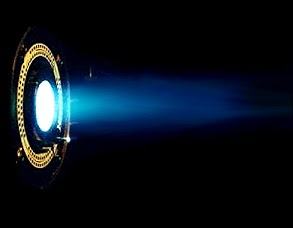Ion thruster

Now that ion thrusters are becoming ever smaller, longer-lasting, and powerful, more missions could be executed in the inner solar system and beyond. Our problem represents an example of a mission that could be executed with current day technology, but it is interesting to consider missions of much greater scale. Perhaps in the not-so-distant future a probe could be launched that visits all the planets of our solar system for a fraction of what it would cost with chemical propellants.

The data we use in our model comes from Jet Propulsion Library’s Horizons database (JPL Solar System Dynamics, n.d.) . This database contains ephemerides (tabulated movements) for various celestial bodies. We were able to generate cartesian coordinates for the position of the solar system starting on May 9th, 2016. The data uses a reference frame with the Sun at the origin.

We will be showcasing the best route for a space probe leaving Earth and visiting other inner solar system planets. First will be a mathematical explanation of our model. Following that will be a toy model to demonstrate the principles being applied. After that we will move into our more complex models and find an optimized route through the inner Solar System. Finally, we will discuss and interpret our results.

## 2. Mathematical model¶

### Variables¶

Our model is based in Newtonian physics. Technically, the only "real" variable we have control over is the 2-vector representing thrust of the probe. However, to simplify the constraints of our model, we chose to have variables for velocity and position in addition to thrust (each of these being 2-vectors as well).

### Constraints¶

As far as constraints are concerned, we have several that are applied at every time step to insure our probe follows the basic laws of kinematics.

• Position: the next position of the probe is the previous position plus the previous velocity times a constant factor
• Velocity: the next velocity of the probe is the previous velocity plus the previous thrust times a constant factor plus the previous force of gravity times a constant factor
• Thrust: the magnitude of thrust at each time step is constrained to be less than a constant value

In addition, we have several constraints that are applied only once.

• Initial Position: the initial position is set to the starting point
• Initial Velocity: the initial velocity is set to the starting velocity
• Final Position: the final position is set to the destination

Our model does not require it, but for our purposes we set initial position to be close to the starting planet and the final position to be the destination planet. By "close" to the starting planet we mean an offset was applied to prevent the gravity of the planet from being too strong for the probe to overcome. Additionally, the initial velocity was specified to match the starting planet's velocity (with respect to the sun). This does not take into account orbital velocity, but we chose to ignore this as it does not greatly impact the final solution given the distance at which we start our probe from the planet.

### Objective Function¶

In our static models as well as our intermediate model, we are either trying to minimize fuel usage or journey duration. However, for our final model, we formulated the objective function to minimize fuel usage and applied $L_2$-regularization to smooth the x- and y-components of the thrust. This regularization makes our solution more feasible because in real life a rocket cannot fire in an arbitrary direction instantly—it takes time to pivot.

Additionally, with our objective function we assumed that fuel use as a function of thrust is quadratic in nature. Theoretically, by conservation of momentum it should be linear—when exhaust velocity is assumed to be constant, doubling the thrust means doubling the momentum of the exhaust and therefore doubling the mass/fuel loss. However, because our thrust can take on both positive and negative values, we would have to take the norm of the 2-vector thrust at every time step and add it up to get the total fuel usage. Instead we chose to square each component of thrust and add them together to obtain the magnitude squared, and it is the sum of these squared magnitudes that we use as our objective value. Making this assumption allows us to code the solution as well as simplifying our model (no square roots are involved).

### Standard Form¶

\begin{aligned} \underset{\mathbf{x}_i,\mathbf{v}_i,\mathbf{u}_i \in \mathbb{R^2}}{\text{minimize}}\qquad& \sum_{i=1}^{n}\mathbf{u}_i\cdot \mathbf{u}_i + \lambda\sum_{i=1}^{n-1} (\mathbf{u}_i - \mathbf{u}_{i+1})^2\\ \text{subject to:}\qquad& \mathbf{u}_i\cdot \mathbf{u}_i -T_{max}^2\le 0 && \forall i \in \{1,\dots,n\}\\ & (\mathbf{x}_{n} - \mathbf{x}_{final}) \cdot (\mathbf{x}_{n} - \mathbf{x}_{final}) - d_{max}^2 \le 0\\ & \mathbf{x}_{1} - \mathbf{x}_{init} = 0\\ & \mathbf{v}_{1} - \mathbf{v}_{init} = 0\\ & \mathbf{x}_{i+1} - \mathbf{x}_{i} - \tau \cdot \mathbf{v}_{i} = 0 && \forall i \in \{1,\dots,n-1\}\\ & \mathbf{v}_{i+1} - \mathbf{v}_{i} - \tau \alpha \left[\frac{\mathbf{u}_{i}}{m} - G \sum_{j=1}^k \frac{M_j}{|\mathbf{r}_{i}^{j} - \mathbf{x}_{i}|^{3/2}} (\mathbf{r}_{i}^j - \mathbf{x}_{i})\right] = 0 &&\forall i \in \{1,\dots,n-1\}\\ & \text{where:}\\ & \qquad \tau \text{ is time step [days]}\\ & \qquad \alpha \text{ is the acceleration conversion (from } \frac{\text{m}}{\text{s}^2} \text{ to } \frac{\text{au}}{\text{day}^2} \text{)}\\ & \qquad m \text{ is the mass of the probe [kg]}\\ & \qquad G \text{ is the universal gravitational constant } \left[\frac{\text{Newton}\cdot\text{au}^2}{\text{kg}^2}\right]\\ & \qquad T_{max} \text{ is the maximum thrust output of the probe [Newtons]}\\ & \qquad n \text{ is the number of time steps [dimensionless]}\\ & \qquad k \text{ is the number of gravity sources [dimensionless]}\\ & \qquad M_j \text{ is the mass of the j}^{\text{th}}\text{ gravity source [kg]}\\ & \qquad \mathbf{r}_{i}^{j} \text{ is the position of gravity source } j \text{ at time step } i\\ & \qquad \mathbf{x}_{init}, \mathbf{x}_{final} \text{ are the initial and ideal final positions}\\ & \qquad \mathbf{v}_{init} \text{ is the initial velocity of the probe}\\ \end{aligned}

Formulated in this manner, the only classification applicable to our particular model is NLP, a non-linear non-convex program. However, two details to note are that a). the problem itself is not necessarily non-convex, just our model of it, and b). all of our constraints could be part of a convex model if our gravity system were simpler. With five different gravity sinks, four of which are moving, the solution space becomes more convoluted and we can no longer assume it is convex. This removes any possiblity of efficient solving using specialized algorithms, and also forces us to keep our number of variables fairly small. However, by using a couple of tricks detailed later, we managed to overcome this and solve the problem on a large scale (~30,000 variables).

## 3. Solution¶

This section is meant to faciliate understanding of the final model in the same way we found the it—starting with the basics and working our way up the ladder of complexity.

### 3.A Proof of Concept¶

To better illustrate the concepts and model we are applying, following are a couple of simplified models optimizing a trajectory through a static gravity system. When trying to optimize such a trajectory, one has to choose what they want to optimize. In our case, we narrowed the list down to whether to find the quickest route or the most fuel-efficient route, so we have provided examples illustrating the pros and cons of both. Below is a contour plot displaying the strength of gravity in the 2-sink gravity system we are using as an example. Before proceeding any further, make a guess as to what you think the optimal trajectory is to get from the start to the destination and then later compare your prediction to what is calculated.

##### Contour Plot of Gravity System¶
In :
using PyPlot

G = .05                     # toy gravitational constant
sink1Pos = [8 1]            # location of gravity sink 1 (x,y)
sink2Pos = [2 -1]           # location of gravity sink 2 (x,y)

initPos = [0 0]             # initial position (x,y)
destPos = [10 0]            # destination position (x,y)

figure(figsize=(14,8))

buffer = .0000001           # removes hole in contour plot

x = -2:.1:12;               # x and y axis limits
y = -4:.1:4;

#***** GENERATE GRAVITY DATA *****#
X = zeros(length(x), length(y))
Y = zeros(length(x), length(y))

for x_ in 1:length(x)
for y_ in 1:length(y)
X[x_,y_] = x[x_]
Y[x_,y_] = y[y_]
end
end

Z =  (((G*(-X + sink1Pos)./((-X + sink1Pos).^2 + (-Y + sink1Pos).^2).^1.5 +
G*(-X + sink2Pos)./((-X + sink2Pos).^2 + (-Y + sink2Pos).^2).^1.5)).^2 +
((G*(-Y + sink1Pos)./((-X + sink1Pos).^2 + (-Y + sink1Pos).^2).^1.5 +
G*(-Y + sink2Pos)./((-X + sink2Pos).^2 + (-Y + sink2Pos).^2).^1.5)).^2) + buffer
Z = log(Z)

#***** PLOT DATA *****#
contourf(X, Y, Z, linspace(-17,2, 100))
plot(sink1Pos, sink1Pos, "mo", sink2Pos, sink2Pos, "mo")
plot(initPos, initPos, "kx")
plot(destPos, destPos, "kx")
legend(["Gravity Sinks"], "upper left")
text(initPos, initPos, " Initial Position")
text(destPos, destPos, " Destination")
text(8.5,-3.1,"Weaker Gravity")
text(2.5,-1.5,"Stronger Gravity")
text(4.1,0,"Equilibrium Point")
axis([-2, 12, -4, 4])
title("Static Gravity Environment")
xlabel("x []")
ylabel("y []")
;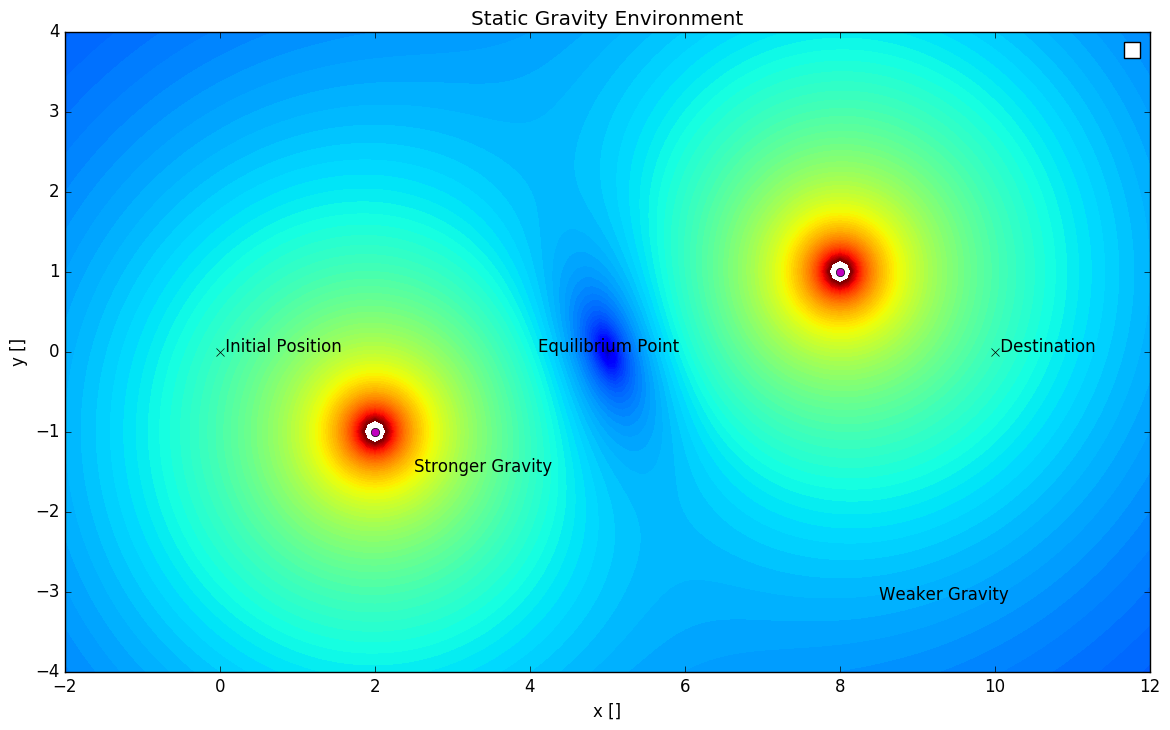#### 3.A.a Minimizing Fuel Consumption¶

One thing to keep in mind with our models, both the time- and fuel-optimal ones, is that we are constraining the probe to arrive at a specific time, i.e. removing the variability of arrival time. The journey is constrained to take the entries of durationSecs as its duration, so the solver is free to take as roundabout a way as it pleases to the goal so long as it minimizes fuel consumption and arrives on time. This can sometimes result in routes that take some interesting detours, as evidenced below.

##### Parameters¶
In :
durationSecs = [60,120,180] # array storing durations of each journey

G = .05                     # toy gravitational constant
sink1Pos = [8 1]            # location of gravity sink 1 (x,y)
sink2Pos = [2 -1]           # location of gravity sink 2 (x,y)

thrustLimit = .01           # limit on magnitude of thrust vector
maxDist = .05               # max distance between destination and probe at end of journey

initPos = [0 0]             # initial position (x,y)
initVelocity = [0 0]        # final position (x,y)

destPos = [10 0]            # destination position (x,y)
;

##### Code¶
In :
using JuMP, Ipopt, PyPlot

lims = round(durationSecs) # number of seconds accounted for in problem

buffer = .0000001 # makes contour plot look nicer

for n in lims
n = Int64(n)
m = Model(solver = IpoptSolver(print_level=0))

#***** DEFINE VARIABLES FOR PROBE POSITIONS, VELOCITIES, AND THRUSTS *****#
@variable(m, AX[1:n,1:2])
@variable(m, AV[1:n,1:2])
@variable(m, AU[1:n,1:2])

#***** DYNAMICS CONSTRAINTS *****#
for i in 1:n-1
# current position is previous + velocity
@constraint(m, AX[i+1,:] .== AX[i, :] + AV[i, :])

# current velocity is previous + thrust + gravity
@NLconstraint(m, AV[i+1, 1] == AV[i, 1] + AU[i, 1] +
G*(-AX[i, 1] + sink1Pos)/((-AX[i, 1] + sink1Pos)^2 + (-AX[i, 2] + sink1Pos)^2)^1.5 +
G*(-AX[i, 1] + sink2Pos)/((-AX[i, 1] + sink2Pos)^2 + (-AX[i, 2] + sink2Pos)^2)^1.5)
@NLconstraint(m, AV[i+1, 2] == AV[i, 2] + AU[i, 2] +
G*(-AX[i, 2] + sink1Pos)/((-AX[i, 1] + sink1Pos)^2 + (-AX[i, 2] + sink1Pos)^2)^1.5 +
G*(-AX[i, 2] + sink2Pos)/((-AX[i, 1] + sink2Pos)^2 + (-AX[i, 2] + sink2Pos)^2)^1.5)
end

#***** KEEP THRUST BELOW CERTAIN VALUE *****#
@constraint(m, (AU[:, 1].^2 + AU[:, 2].^2) .<= thrustLimit^2)

#***** START CONSTRAINTS *****#
@constraint(m, AX[1, :] .== initPos)
@constraint(m, AV[1, :] .== initVelocity)

#***** RENDEZVOUS CONSTRAINT *****#
@constraint(m, sum((AX[n, :] - destPos).^2) <= maxDist^2)

#***** MINIMIZE FUEL USAGE *****#
@objective(m, Min, sum(AU[:,1].^2 + AU[:,2].^2))

solve(m)

AX = getvalue(AX); AU = getvalue(AU)

x = -2:.1:12;
y = -4:.1:4;

X = zeros(length(x), length(y))
Y = zeros(length(x), length(y))

for x_ in 1:length(x)
for y_ in 1:length(y)
X[x_,y_] = x[x_]
Y[x_,y_] = y[y_]
end
end

Z =  (((G*(-X + sink1Pos)./((-X + sink1Pos).^2 + (-Y + sink1Pos).^2).^1.5 +
G*(-X + sink2Pos)./((-X + sink2Pos).^2 + (-Y + sink2Pos).^2).^1.5)).^2 +
((G*(-Y + sink1Pos)./((-X + sink1Pos).^2 + (-Y + sink1Pos).^2).^1.5 +
G*(-Y + sink2Pos)./((-X + sink2Pos).^2 + (-Y + sink2Pos).^2).^1.5)).^2) + buffer

Z = log(Z)

figure(figsize=(14,8))

contourf(X, Y, Z, linspace(-17,2, 100))
plot(AX[:, 1], AX[:, 2], "k-", sink1Pos, sink1Pos, "mo", sink2Pos, sink2Pos, "mo")
plot(initPos, initPos, "kx")
plot(destPos, destPos, "kx")
grid("on")
axis([-2, 12, -4, 4])
title(string("Minimize Fuel Use: ", n, " seconds"))
text(-1.5,-3.5,string("Objective value is: ", getobjectivevalue(m)))
xlabel("x []")
ylabel("y []")
end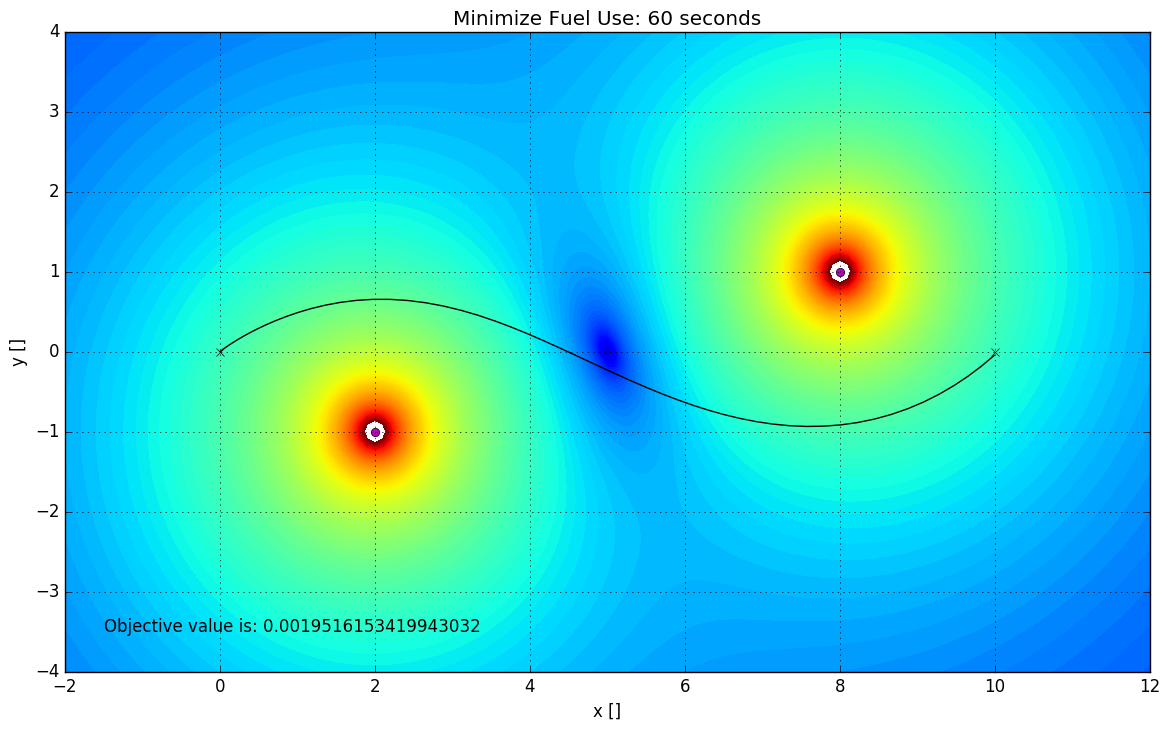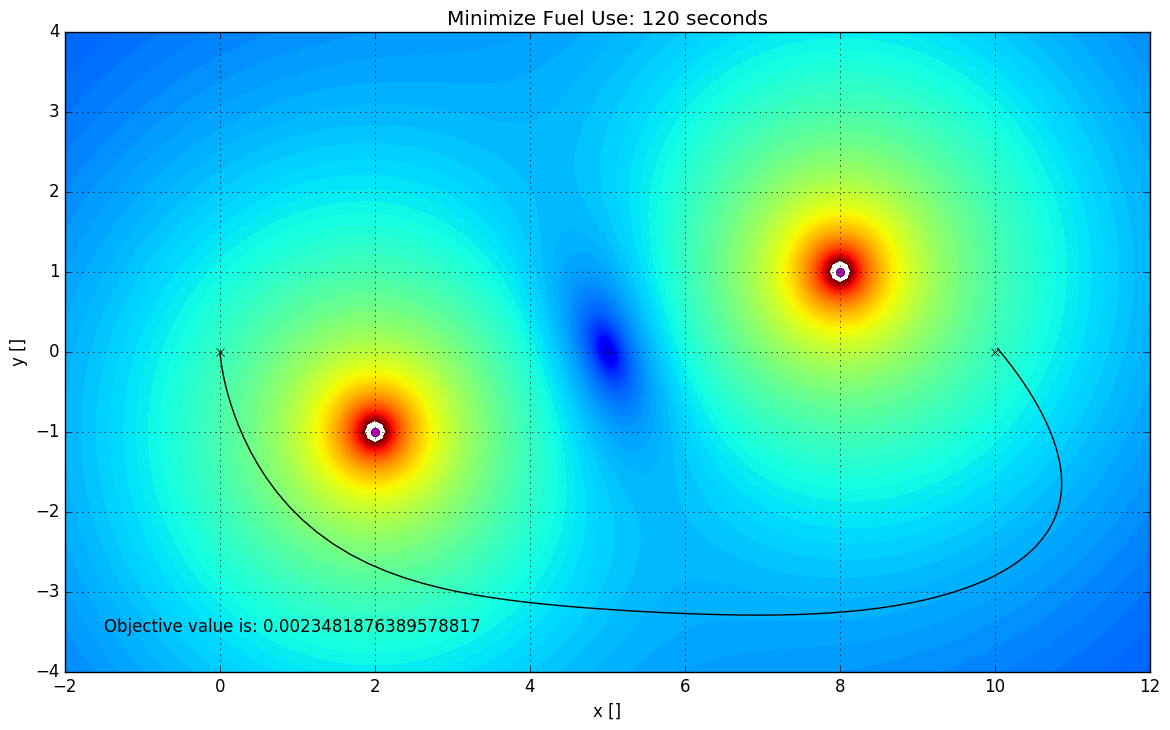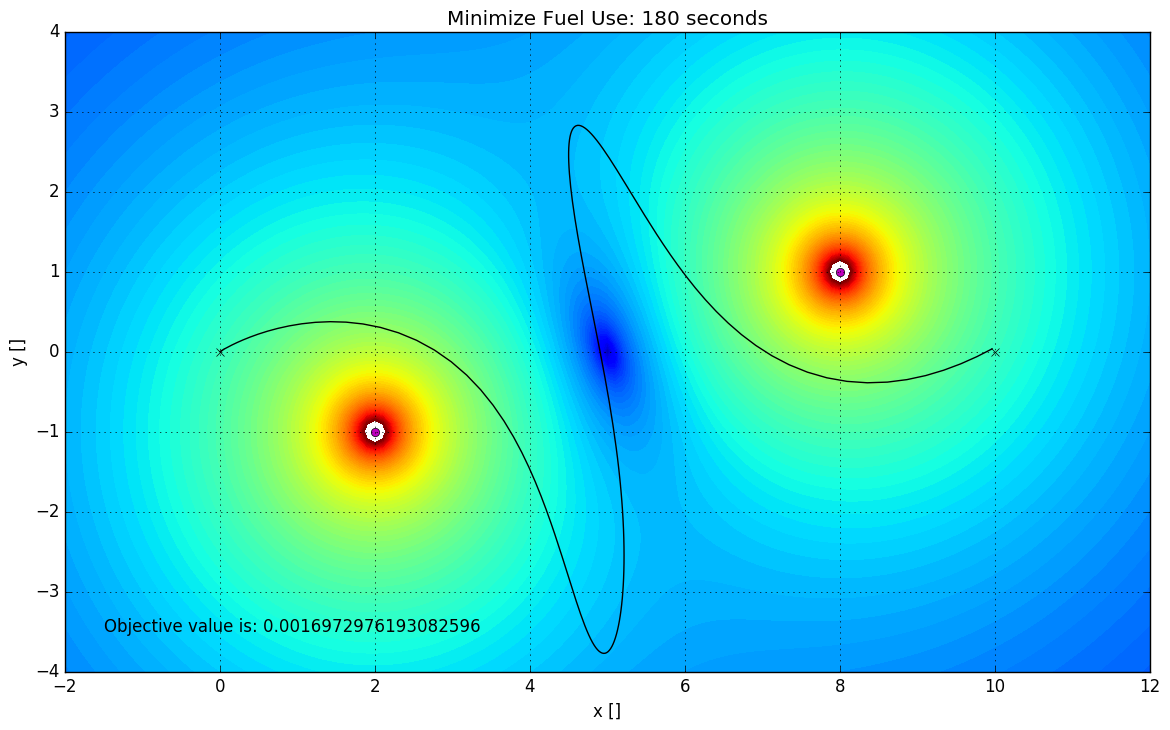Notice that just by changing a single thing, the amount of time taken for the journey, the most fuel-efficient route can change drastically. The optimal route for $t = 60 \text{ seconds}$ is what you probably predicted. Such a route strikes a good balance between the amount of fuel used and the amount of time "wasted" on detours. An interesting thing to notice about these plots is the objective values of each. The route for $t = 60$ has a value of $\approx0.001952$ (no meaningful units) while the route for $t = 180$ has a value of $\approx0.001697$, indicating that the longer path is actually more fuel-efficient.

This can be explained by the way the $t = 180$ route uses gravity. It rides the gravity well of the first sink in the same way a penny rides a spiral wishing well, circling rather than resisting the pull "downward." After it reaches a certain point, it uses its thrusters to give it a little push out of the well and then flies along the gravitational equilibrium before taking the plunge and riding the other well to its destination.

The $t = 60$ route, on the other hand, takes a more direct approach, following an $S$-curve to utilize gravity to a certain extent but relying more heavily on thrusters to get the probe where it needs to be quicker. In a sense it fights through the waves rather than ride them, and this is evident in the plots above.

In the more complicated, dynamic gravity systems we use for optimizing a probe's trajectory in the inner solar system, striking a balance between time and fuel factors heavily into the way we formulate our model. Another tool we used to perform this balancing act was the minimization of journey duration.

#### 3.A.b Minimize Journey Duration¶

For this model, on the other hand, we are trying to get the probe to its destination as fast as possible. To be clear, we would like to minimize t where time t is the first time at which the distance between the probe and the destination is less than the maxDist parameter. However, there is not a simple, standard way to constrain t thusly, and we were forced to improvise a little to optimize for trip duration.

In the end, to minimize the time taken we ended up constructing an objective function that strongly penalizes being far away from the destination, encouraging solutions that move toward the destination rapidly. We implemented this by squaring the distance between the destination and the probe and adding it up for all the time steps.

$$f_{\text{obj}} = \sum_{i=1}^{n}(\mathbf{x}_i-\mathbf{x_{final}})\cdot(\mathbf{x}_i-\mathbf{x_{final}})$$
##### Parameters¶
In :
durationSecs = [75,60,45]   # array storing durations of each journey

G = .05                     # toy gravitational constant
sink1Pos = [8 1]            # location of gravity sink 1 (x,y)
sink2Pos = [2 -1]           # location of gravity sink 2 (x,y)

thrustLimit = .01           # limit on magnitude of thrust vector
maxDist = .05               # max distance between destination and probe after journey

initPos = [0 0]             # initial position (x,y)
initVelocity = [0 0]        # final position (x,y)

destPos = [10 0]            # destination position (x,y)
;

##### Code¶
In :
using JuMP, Ipopt, PyPlot

lims = round(durationSecs) # number of seconds accounted for in problem

buffer = .0000001 # makes contour plot look nicer

for n in lims
n = Int64(n)
m = Model(solver = IpoptSolver(print_level=0))

#***** DEFINE VARIABLES FOR PROBE POSITIONS, VELOCITIES, AND THRUSTS *****#
@variable(m, AX[1:n,1:2])
@variable(m, AV[1:n,1:2])
@variable(m, AU[1:n,1:2])

#***** DYNAMICS CONSTRAINTS *****#
for i in 1:n-1
# current position is previous + velocity
@constraint(m, AX[i+1,:] .== AX[i, :] + AV[i, :])

# current velocity is previous + thrust + gravity
@NLconstraint(m, AV[i+1, 1] == AV[i, 1] + AU[i, 1] +
G*(-AX[i, 1] + sink1Pos)/((-AX[i, 1] + sink1Pos)^2 + (-AX[i, 2] + sink1Pos)^2)^1.5 +
G*(-AX[i, 1] + sink2Pos)/((-AX[i, 1] + sink2Pos)^2 + (-AX[i, 2] + sink2Pos)^2)^1.5)
@NLconstraint(m, AV[i+1, 2] == AV[i, 2] + AU[i, 2] +
G*(-AX[i, 2] + sink1Pos)/((-AX[i, 1] + sink1Pos)^2 + (-AX[i, 2] + sink1Pos)^2)^1.5 +
G*(-AX[i, 2] + sink2Pos)/((-AX[i, 1] + sink2Pos)^2 + (-AX[i, 2] + sink2Pos)^2)^1.5)
end

#***** KEEP THRUST BELOW CERTAIN VALUE *****#
@constraint(m, (AU[:, 1].^2 + AU[:, 2].^2) .<= thrustLimit^2)

#***** START CONSTRAINTS *****#
@constraint(m, AX[1, :] .== initPos)
@constraint(m, AV[1, :] .== initVelocity)

#***** RENDEZVOUS CONSTRAINT *****#
@constraint(m, sum((AX[n, :] - destPos).^2) <= maxDist^2)

#***** MINIMIZE TIME (APPROX.) *****#
@objective(m, Min, sum((AX[:,1]-destPos).^2 + (AX[:,2]-destPos).^2))

solve(m)

AX = getvalue(AX)

x = -2:.1:12;
y = -4:.1:4;

X = zeros(length(x), length(y))
Y = zeros(length(x), length(y))

for x_ in 1:length(x)
for y_ in 1:length(y)
X[x_,y_] = x[x_]
Y[x_,y_] = y[y_]
end
end

Z =  (((G*(-X + sink1Pos)./((-X + sink1Pos).^2 + (-Y + sink1Pos).^2).^1.5 +
G*(-X + sink2Pos)./((-X + sink2Pos).^2 + (-Y + sink2Pos).^2).^1.5)).^2 +
((G*(-Y + sink1Pos)./((-X + sink1Pos).^2 + (-Y + sink1Pos).^2).^1.5 +
G*(-Y + sink2Pos)./((-X + sink2Pos).^2 + (-Y + sink2Pos).^2).^1.5)).^2) + buffer

Z = log(Z)

figure(figsize=(14,8))

contourf(X, Y, Z, linspace(-17,2, 100))
plot(AX[:, 1], AX[:, 2], "k-", sink1Pos, sink1Pos, "mo", sink2Pos, sink2Pos, "mo")
plot(initPos, initPos, "kx")
plot(destPos, destPos, "kx")
grid("on")
axis([-2, 12, -4, 4])
title(string("Minimize Duration: ", n, " seconds"))
xlabel("x []")
ylabel("y []")
end